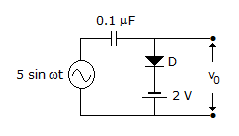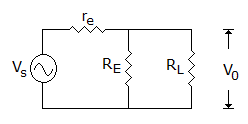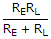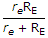# Electronics and Communication Engineering - Analog Electronics

16.

The phase angle of lead circuit is

 A. between 0 and -90° B. between 0 and +90° C. 90° D. more than 90°

Explanation:

No answer description available for this question. Let us discuss.

17.

A generator with an internal resistance of 600 Ω drives an amplifier whose input resistance is 400 Ω. The generator's open circuit voltages is 1 mV; the amplifier develops 100mV across an 8kΩ load. The power gain is

 A. 125 B. 250 C. 1125 D. 3125

Explanation:

No answer description available for this question. Let us discuss.

18.

The circuit of figure isA. clipper, sine wave clipped at - 2 V B. clamper C. clamper, sine wave clamped at zero V D. clipper, sine wave clipped at + 2 V

Explanation:

No answer description available for this question. Let us discuss.

19.

The upper end of the d.c. load line is called the __________ point and the lower end is the __________ point.

 A. cut off, saturation B. saturation, active C. saturation, cut off D. active saturation

Explanation:

No answer description available for this question. Let us discuss.

20.

In figure output impedance of amplifier isA. RE B.C.D. re + RE